2022 AL Cy Young Odds
+500
5 to 1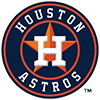Justin Verlander
12.8% implied probability

+600
6 to 1Kevin Gausman
11.0% implied probability

+750
7.5 to 1Gerrit Cole
9.1% implied probability

+900
9 to 1Shohei Ohtani
7.7% implied probability

+900
9 to 1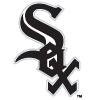Dylan Cease
7.7% implied probability

+1000
10 to 1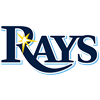Shane McClanahan
7.0% implied probability

+1100
11 to 1Alek Manoah
6.4% implied probability

+1600
16 to 1Nestor Cortes
4.5% implied probability

+2500
25 to 1Shane Bieber
3.0% implied probability

+2500
25 to 1Lucas Giolito
3.0% implied probability

+4000
40 to 1Noah Syndergaard
1.9% implied probability

+4000
40 to 1Tarik Skubal
1.9% implied probability

+4000
40 to 1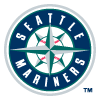Logan Gilbert
1.9% implied probability

+4500
45 to 1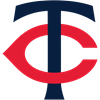Joe Ryan
1.7% implied probability

+5000
50 to 1Michael Kopech
1.5% implied probability

+5000
50 to 1Patrick Sandoval
1.5% implied probability

+6000
60 to 1Frankie Montas
1.3% implied probability

+7000
70 to 1Robbie Ray
1.1% implied probability

+7000
70 to 1Framber Valdez
1.1% implied probability

+8500
85 to 1Luis Garcia
0.9% implied probability

+8500
85 to 1Paul Blackburn
0.9% implied probability

+10000
100 to 1Luis Severino
0.8% implied probability

+12000
120 to 1Garrett Whitlock
0.6% implied probability

+12000
120 to 1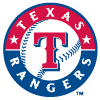Martin Perez
0.6% implied probability

+12000
120 to 1Drew Rasmussen
0.6% implied probability

No changes have been recorded yet.
+12000
120 to 1Triston McKenzie
0.6% implied probability

+15000
150 to 1Jordan Montgomery
0.5% implied probability

+15000
150 to 1Aroldis Chapman
0.5% implied probability

+15000
150 to 1Michael King
0.5% implied probability

+15000
150 to 1Nathan Eovaldi
0.5% implied probability

+15000
150 to 1Lance McCullers
0.5% implied probability

+15000
150 to 1Lance Lynn
0.5% implied probability

+15000
150 to 1Sonny Gray
0.5% implied probability

+15000
150 to 1Eduardo Rodriguez
0.5% implied probability

+15000
150 to 1Jose Berrios
0.5% implied probability

+25000
250 to 1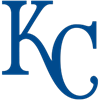Zack Greinke
0.3% implied probability

+25000
250 to 1Corey Kluber
0.3% implied probability

+25000
250 to 1Shane Baz
0.3% implied probability

+25000
250 to 1Yusei Kikuchi
0.3% implied probability

+25000
250 to 1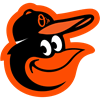Bruce Zimmermann
0.3% implied probability

+25000
250 to 1Chris Sale
0.3% implied probability

+25000
250 to 1Jose Urquidy
0.3% implied probability

+25000
250 to 1Liam Hendriks
0.3% implied probability

+25000
250 to 1Jameson Taillon
0.3% implied probability

+50000
500 to 1Jake Odorizzi
0.2% implied probability

+50000
500 to 1Hyun Jin Ryu
0.2% implied probability

+50000
500 to 1Aaron Civale
0.2% implied probability

+50000
500 to 1Emmanuel Clase
0.2% implied probability

+50000
500 to 1Jon Gray
0.2% implied probability

+50000
500 to 1Casey Mize
0.2% implied probability

+50000
500 to 1Zach Plesac
0.2% implied probability

+50000
500 to 1Kenta Maeda
0.2% implied probability

+50000
500 to 1Chris Flexen
0.2% implied probability

+50000
500 to 1Brady Singer
0.2% implied probability

+50000
500 to 1Marco Gonzales
0.2% implied probability